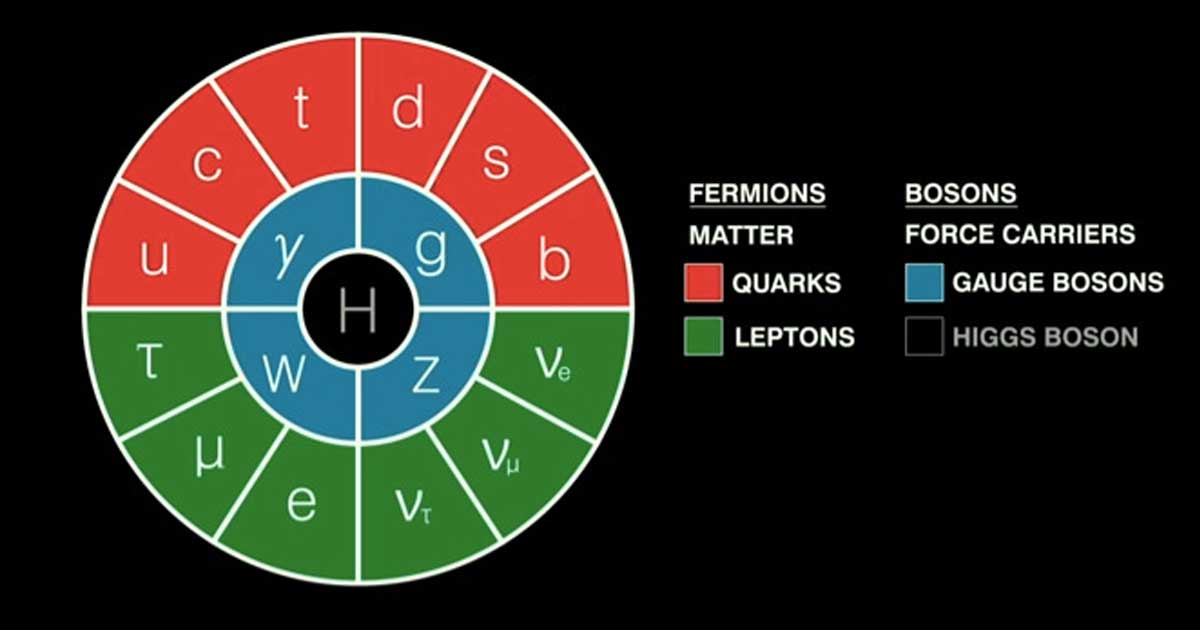Mass (m) is the measure of a bodies energy content, a measure of how much energy is needed to accelerate an object, and a measure of the extent to which a body curves spacetime. The affects of mass give bodies “gravity” and “weight”. There are a few different ways to measure mass, but all can be understood through the lens of Newton and Einstein. Mass (potential energy) is understood as equivalent to energy (kinetic energy) using the speed of light as a conversion factor (E=mc2.). The fact that mass and energy can be conserved each other, means we can refer to both as simply mass-energy. All mass types can understood as the amount of force needed to accelerate an object (f=ma), except for quantum mass which is the frequency of energy in Planck units (E=hf).

## What is Light?We explain “light,” both as electromagnetic radiation within a visible portion of the electromagnetic spectrum, and as electromagnetic energy carried by photons.

## The Standard Model (of Particle Physics) ExplainedWe explain the standard model of particle physics in simple terms for non-experts using videos, facts, and bullet points.